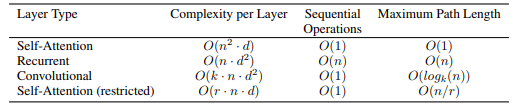# Self-Attention and Positional Encoding

self.P[:, :, 1::2] = torch.cos(X) in section 10.6.3 breaks if encoding_dim is odd number.

This chapter says that the the maximum path length of CNN is \mathcal{O}(n/k). I think it should be \mathcal{O}(log_k n)(as below)This blog also talks about the maximum path length:https://medium.com/analytics-vidhya/transformer-vs-rnn-and-cnn-18eeefa3602b

@Zhaowei_Wang O(log_k(n)) is the case of dilated convolutions, while the chapter discusses regular convolutions.